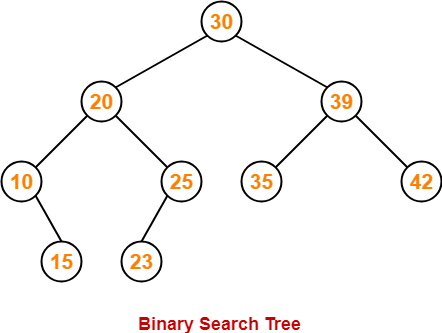# Construct BST from the given pre-order traversal

## Introduction

Before beginning with this question, let’s recap what a BST is and its various types of traversals.

BST is a unique binary tree where each node contains a value greater than all the values present in the left subtree and smaller than or equal to the values present in the right subtree.

Example -Source

Pre-order Traversal of a Binary Tree - In this, we first traverse the root, then left subtree, and then right subtree. Pre-order Traversal of the above tree is: 30 20 10 15 25 23 39 35 42

Inorder Traversal of a Binary Tree - We first traverse the left subtree, root, and right subtree. Inorder Traversal of the above tree is: 10 15 20 23 25 30 35 39 42

Postorder Traversal of a Binary Tree - In this, we first traverse the left subtree, then the right subtree, and then the root. Postorder Traversal of the above tree is: 15 10 23 25 20 35 42 39 30

## Problem Statement

Construct a BST from the given pre-order traversal of a Binary Search Tree (BST). Assume BST has a unique value at each node.

Example:

I/P - [30 20 10 15 25 23 39 35 42]

O/P - Above Tree

## Approach

We know that the inorder traversal of a BST is always sorted. Therefore on sorting the pre-order traversal of a BST, we will get the inorder traversal of that BST. Since from the previous article, we know we can construct the BST using inorder and pre-order traversal of a tree in O(N) time.

So we will follow the same approach from here onwards.

## Code in C++

``````#include<bits/stdc++.h>
using namespace std;

class TreeNode{
public:
int value;
TreeNode *left , *right;
TreeNode(int value){
this->value = value;
left = NULL;
right = NULL;
}
};

// In this hashmap(key,value)
// key = value of a node in BST
// value = index at which this value is present inside the preorder traversal array
unordered_map<int,int> indexes;

TreeNode* constructTreeFromPreorderInorder(vector<int>& preorder,vector<int>& inorder,int preStart,int preEnd,int inStart,int inEnd){

if(preStart>preEnd){
return NULL;
}

int rootValue = preorder[preStart];
TreeNode* root = new TreeNode(rootValue);
if(preStart==preEnd){
// only 1 node is present in the tree
return root;
}

// finding the index of the root in the inorder traversal array
int rootIndexInorder = indexes[rootValue];

// finding the number of nodes on the left subtree
int totalNodesOnLeft = (rootIndexInorder - inStart);

TreeNode* leftTree = constructTreeFromPreorderInorder(
preorder,
inorder,
preStart+1,
preStart+totalNodesOnLeft,
inStart,
inStart + totalNodesOnLeft - 1
);

TreeNode* rightTree = constructTreeFromPreorderInorder(
preorder,
inorder,
preStart+totalNodesOnLeft + 1,
preEnd,
inStart + totalNodesOnLeft +1,
inEnd
);

root->left = leftTree;
root->right = rightTree;
return root;
}

TreeNode* bstFromPreorder(vector<int>& preorder) {
int n = preorder.size();

// Since
vector<int> inorder(n);
for(int i=0;i<n;i++){
inorder[i] = preorder[i];
}
sort(inorder.begin(),inorder.end());

// Storing the indexes of the inorder traversal
for(int i=0;i<n;i++){
indexes[inorder[i]] = i;
}

TreeNode* root = constructTreeFromPreorderInorder(preorder,inorder,0,n-1,0,n-1);
return root;
}

void printInorder(TreeNode* root){
if(root==NULL){
return;
}
printInorder(root->left);
cout<<root->value<<" ";
printInorder(root->right);
}

int main()
{

// 	    INPUT TREE
//          30
//        /     \
//      20       39
//      /  \    /  \
//    10    25 35  42
//      \   /
//      15  23

// Pushing the preorder traversal of the above tree
vector<int> preorder;
preorder.push_back(30);
preorder.push_back(20);
preorder.push_back(10);
preorder.push_back(15);
preorder.push_back(25);
preorder.push_back(23);
preorder.push_back(39);
preorder.push_back(35);
preorder.push_back(42);

// Getting the Tree Constructed from it
TreeNode* root = bstFromPreorder(preorder);

cout<<"Inorder of the above tree constructed is : "<<endl;
printInorder(root);
} ``````

### Output

``````Inorder of the above tree constructed is :
10 15 20 23 25 30 35 39 42 ``````

### Time Complexity

We are sorting the pre-order traversal to get the inorder traversal. Therefore T(n) = O(N log N), where n = the total number of nodes in a tree.

### Space Complexity

We make an extra array to store inorder traversal. Therefore space complexity is O(n), where n = Total number of nodes in a tree.

1. What is a BST (Binary Search Tree)?
BST is a binary tree in which every node of the tree should satisfy these two properties:
1. All the nodes in the left subtree should have lesser values than the value at the current node.
2. All the nodes in the right subtree should have values that are either equal to or more than the value at the current node.

2. How is inorder traversal of a BST always sorted?
In an inorder traversal, since we first travel on the left subtree (which has values lower than the current node), then root and the right subtree (which has values greater than or equal to values present in the right subtree). So we are traversing the tree in a sorted manner:

Left Subtree → Root → Right subtree.

## Key Takeaways

This article taught us how to find the inorder traversal of a BST from its pre-order traversal. We also learned how to construct the BST from pre-order and inorder traversal.

Since Binary Search Tree is frequently asked in interviews, we recommend you practice more problems based on Binary Search Trees on CodeStudio.